## Saturday, 20 October 2012

### Calculating Percentage Solutions

For some reason percentage solution calculation cause students some problems. However, hopefully, once you have been through this blog post you should have a good understanding of percentage solutions, and how to do the calculations.

If you want to practice your 'science maths' than have a look at: Maths4Biosciences.com

If you are having problems with percentage solution calculations then you might like to check out the Percentage Solutions course.

What are percentage solutions?

A percentage solution is an amount or volume of something per 100 ml or 100 g of a solution. It is as simple as that. It is a percentage.

Why are they used?

Percentage solutions are just a convenient and easy way to record solution concentrations. One advantage of percentage solutions is that you don’t need to know anything about the compound in terms of molecular weight as all you need is the percentage of the required solution.

Why are there three types of percentage solutions?

This is slightly difficult to explain. However, there are three types of percentage solution:
• Percentage weight by volume (w/v)
• Percentage volume by volume (v/v)
• Percentage weight by weight (w/w)
The percentage weight by volume (w/v) is the number of grams of compound per 100 ml of solution. This type of percentage solution tends to be used when describing the amount of some powder made up in a solution. So, for example, 5 g of a powder made up to a final volume of 100 ml would be a 5% (w/v) solution. Likewise, 2.5 g of powder made up to 50 ml would also be a 5% (w/v) solution as you would have 5 g in 100 ml.

The percentage volume by volume (v/v) is the number of ml of some liquid per 100 ml of the solution. This type of percentage solution is usually used to describe a solution that is made by mixing two liquids. So, for example, 5 ml of a liquid made up to a final volume of 100 ml would be a 5% (v/v) solution. Likewise, 2.5 ml of a liquid made up to 50 ml would also be a 5% (v/v) solution as you would have 5 ml in 100 ml.

Finally, the percentage weight by weight (w/w). This one is a little more difficult to understand, but the principles as explained above are still true. A percentage weight by weight (w/w) solution can be a weight of a powder or a liquid made up in a solution to a final weight of the solution. So, for example, 5 g of a powder (or a liquid) made up in a solution that has a final weight of 100 g would be a 5% (w/w) solution. Likewise, 2.5 g of a liquid or powder made up to give a solution that weighed 50 g would also be a 5% (w/w) solution as you would have 5 g of the powder or liquid in 100 g of a solution.

If you are having problems with percentage solution calculations then you might like to check out the Percentage Solutions.

## Tuesday, 16 October 2012

### 3d Structure of the GDP G-protein alpha subunit

The movie below shows the 3d structure of a guanosine diphosphate (GDP) bound G protein alpha-subunit.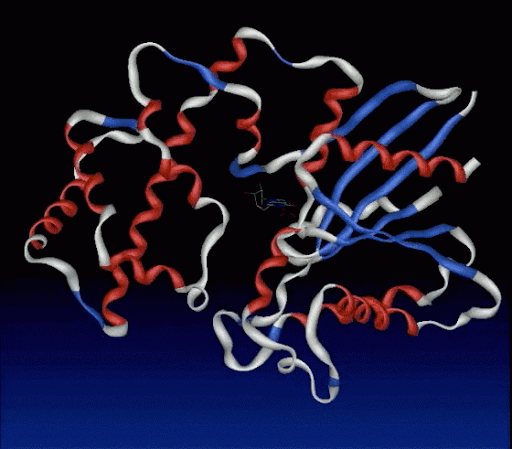The small green molecule in the middle is the bound GDP.

http://www.rcsb.org/pdb/cgi/explore.cgi?pdbId=1TAG

### Bovine Rhodopsin

The structure of a G-protein coupled receptor is often shown as: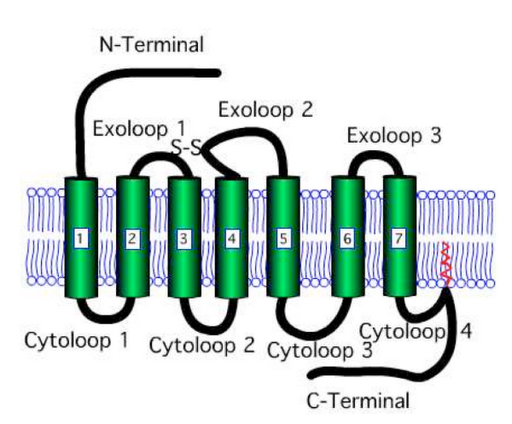However, the crystal structure of bovine rhodopsin shows that the seven transmembrane spanning domains actually form a tight core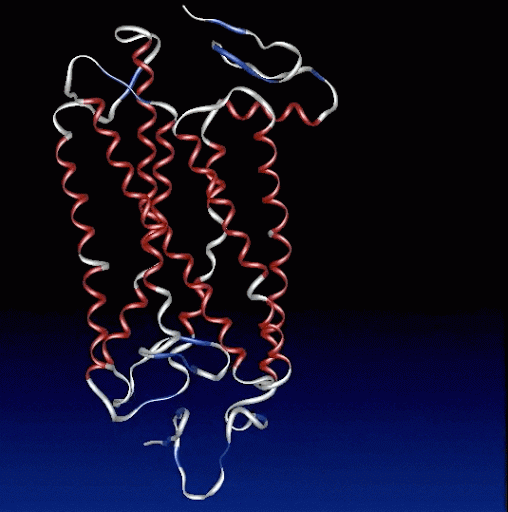J. Mol. Biol. (2004) 342, 571–583 http://www.rcsb.org/pdb/cgi/explore.cgi?pdbId=1U19

### 3d Structure - β2AR-T4L

The structure of a G-protein coupled receptor is often shown as:Which shows a receptor with the N-terminal outside the cell, seven transmembrane spanning domains, 3 exoloops, 4 cytoloops and a C terminal inside the cell.

However, it is actually more compact with the seven transmembrane spanning domains forming a tight core.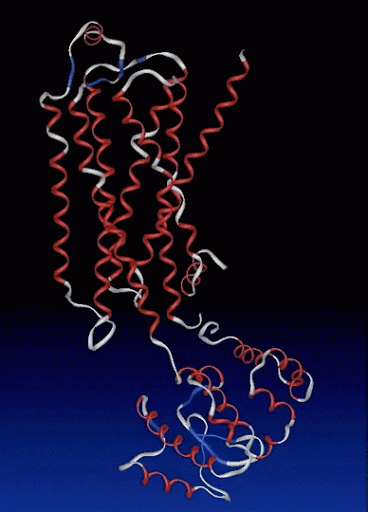Lower part = T4-lysozyme in place of cyto 3 Small green molecules = cholesterol; can just make out bound ligand in pocket
Science. 2007 318(5854): 1258–1265. http://www.rcsb.org/pdb/cgi/explore.cgi?pdbId=2RH1

The following animation shows αGs interacting with adenylyl cyclase.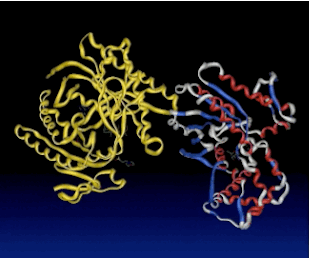The animation is also available as a slightly larger video....

### GPCR movement of TM3 and 6

I have been asked for access to a number of the movies I used in the lectures.

The following animation shows the proposed movements of transmembrane spanning domains 3 and 6 for rhodopsin and β2 adrenergic receptor.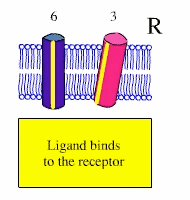It should be noted that other GPCR may use different methods.